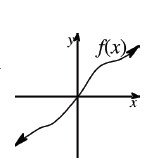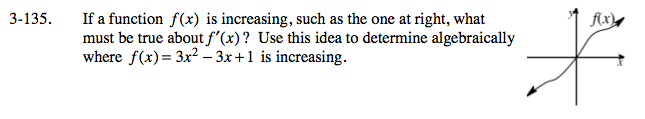### Home > CALC > Chapter 3 > Lesson 3.3.4 > Problem3-135

3-135.
1. If a function f(x) is increasing, such as the one below, what must be true about f ′(x) ? Use this idea to determine algebraically where f(x) = 3x2 − 3x + 1 is increasing. Homework Help ✎If f(x) is increasing, then slopes are positive so f '(x) > 0.

Use the Power Rule to find f '(x).

Solve the inequality: f '(x) > 0.